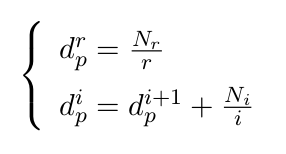# Department of Mathematics and Computer Science

www.univ-soukahras.dz/en/dept/mi

Module: Initiation à la recherché et code de déontologie

1. Information
2. Questions

## Writing Equations using Latex (5)

 2 votes What's the latex code for the following Equation:Asked on 19:29, Monday 16 May 2016 By Imed BOUCHRIKA In Initiation à la recherché et code de déontologie

 0 votes \begin{equation} \left \{ \begin{array}{r c l } d_p^r = \frac{N_r}{r} \\ \\ d_p^i = d_p^{i+1} + \frac{N_i}{i} \end{array} \right . \end{equation} Answered on 22:09, Tuesday 17 May 2016 by Hanen Rouainia(322 points) In Initiation à la recherché et code de déontologie

 0 votes \begin{equation} \left \{ \begin{array}{l} d_p^r = \frac {N_r}{r} \\ d_p^i = d_p^{i+1} + \frac{N_i}{i} \end{array} \right . \end{equation} Answered on 15:57, Friday 27 May 2016 by amirat abdallah(257 points) In Initiation à la recherché et code de déontologie